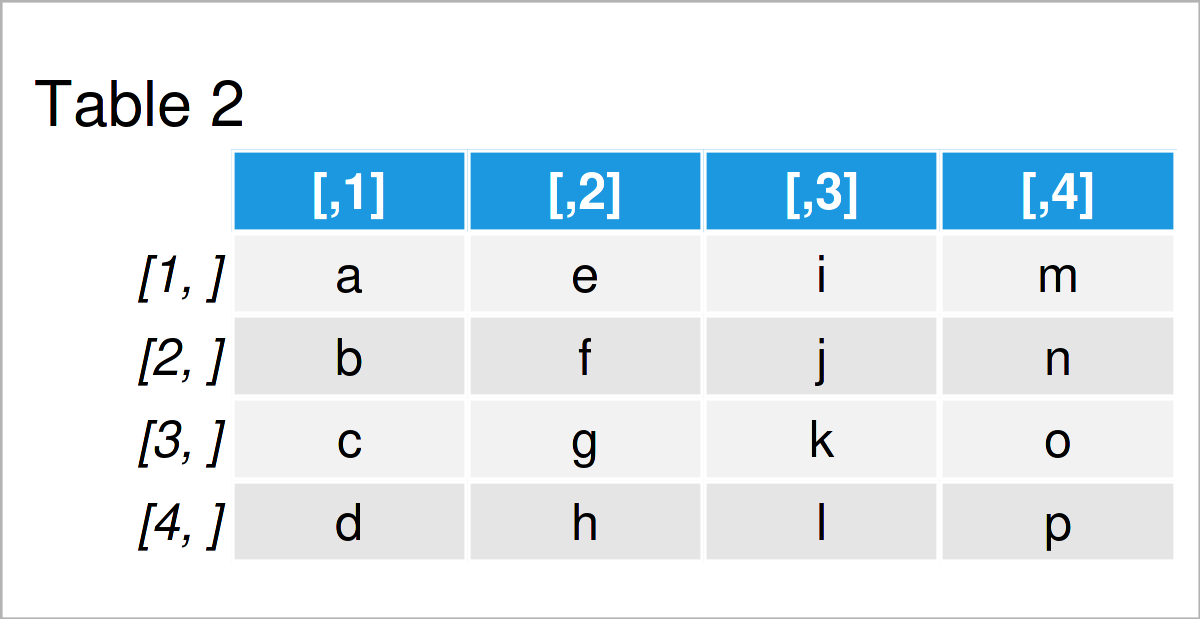# Create List of Matrices in R (Example)

In this article you’ll learn how to store multiple matrices in a list object in R.

The article will consist of this content:

So let’s take a look at some R codes in action…

## Creation of Example Data

We’ll use the following data as basement for this R programming tutorial:

```mat1 <- matrix(1:15, ncol = 3)             # Create first example matrix
mat1                                       # Print first example matrix```Table 1 shows the structure of our first example matrix: It contains five rows and three integer columns.

Let’s create a second matrix object:

```mat2 <- matrix(letters[1:16], ncol = 4)    # Create second example matrix
mat2                                       # Print second example matrix```The output of the previous R syntax is shown in Table 2: Another matrix containing character letters.

## Example: Create List of Matrices Using list() Function

This example demonstrates how to combine multiple matrix objects in a single list.

For this task, we can apply the list function as shown below:

```my_list <- list(mat1, mat2)                # Create list
my_list                                    # Print list
# []
#      [,1] [,2] [,3]
# [1,]    1    6   11
# [2,]    2    7   12
# [3,]    3    8   13
# [4,]    4    9   14
# [5,]    5   10   15
#
# []
#      [,1] [,2] [,3] [,4]
# [1,] "a"  "e"  "i"  "m"
# [2,] "b"  "f"  "j"  "n"
# [3,] "c"  "g"  "k"  "o"
# [4,] "d"  "h"  "l"  "p"```

As you can see, each matrix has been stored as a separate list element.

## Video & Further Resources

Have a look at the following video on my YouTube channel. In the video, I illustrate the R programming syntax of this page in a live session in RStudio.

Please accept YouTube cookies to play this video. By accepting you will be accessing content from YouTube, a service provided by an external third party.If you accept this notice, your choice will be saved and the page will refresh.

In addition, you might want to read the related articles on this website. You can find some interesting articles below:

In summary: In this article, I have explained how to combine multiple matrices in a list object in R programming. If you have additional questions, let me know in the comments. Furthermore, please subscribe to my email newsletter in order to get updates on new tutorials.

Subscribe to the Statistics Globe Newsletter